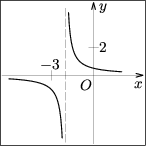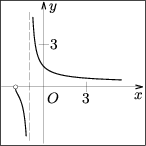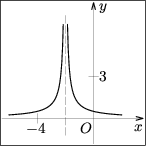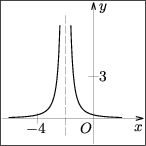CTU FCE Department of Mathematics
Thákurova 7, 166 29 Praha 6
Phone: +420 / 224 354 390

## Entrance Exam from Mathematics – online testing

There is a multiple choice of 5 answers in each of the 15 assignments of the following online test. There is always only one correct answer.

Please mark the answer you consider correct in the form on the left side.

If you click on the answer, its background should change to black. The answers can be marked in any internet browser that supports CSS pseudo class :checked. If you click on the answer and it is not marked (there is no black background), please try another internet browser. Even if the answers are not visually marked after you click on them, they are selected and will be processed when you send the test back for evaluation.

Time allotted for test is 70 minutes. When the time is up, the test is terminated and evaluated. You can finish the test before the end of trial period. Remaining time is shown in the right top hand corner of the page.

You need to have javascript enabled.

 a b c d e
1 point
 1. The line ${ax-5y+c=0}$ and the line $AB$, where $A[-2,\,-1]$, $B[3,\,1]$, are identical if and only if a) $a=5\lland c=5$ b) $a=2\lland c=1$ c) $a=-5\lland c=-15$ d) $a=-2\lland c=-9$ e) $a=2\lland c=-1$
 a b c d e
2 points
 2. An equation of the ellipse with the axes parallel to the coordinate axes and tangent to both coordinate axes at the points ${M[-4,\,0]}$, ${N[0,\,3]}$ is a) ${3x^2+4y^2+36x-48y+96=0}$ b) ${9x^2+16y^2+72x-96y+144=0}$ c) ${3x^2+4y^2+18x-20y+24=0}$ d) ${x^2+2y^2+7x-10y+12=0}$ e) ${9x^2+16y^2+39x-52y+12=0}$
 a b c d e
1 point
 3. The term $a_4$ of the sequence $(a_n)_{n=1}^{\infty}$, that is given by the recurrent formula ${a_{n+1}=(n+1)a_n-5}$ and by the term ${a_1=0}$, is a) $-20$ b) $-430$ c) $-48$ d) $-85$ e) $-80$
 a b c d e
1 point
 4. If ${\cos2\alpha=-1}$, then a) $\tg\alpha=0$ b) $\tg\alpha$ is undefined c) $\tg\alpha=\sqrt3$ d) $\tg\alpha=-1$ e) $\tg\alpha=1$
 a b c d e
2 points
 5. In the cube $ABCDEFGH$ with the side $a$ denote $L$ as a midpoint of side $EH$. The volume of pyramid $BCDL$ is a) $\dfrac{a^3}2$ b) $\dfrac{a^3}{3\sqrt2}$ c) $\dfrac{a^3}3$ d) $\dfrac{a^3}6$ e) $\dfrac{a^3}8$
 a b c d e
1 point
 6. Find the set of all solutions of the inequality ${\dfrac1{|2x+3|}<1}$ with the unknown ${x\in\R}$. a) $(-\infty,\,2\rangle$ b) $(-1,\,\infty)$ c) $(-\infty,\,-2)\cup(-1,\,\infty)$ d) $(-2,\,-\frac32)\cup(-\frac32,\,2)$ e) $(-\infty,\,-\frac32)\cup(-\frac32,\,\infty)$
 a b c d e
1 point
 7. The algebraic form of the complex number ${z=(1+2\i)[\cos(\frac14\pi)-\i\sin(\frac14\pi)]}$ is a) $\frac{3\sqrt2}2+\frac{\sqrt2}2\,\i$ b) $3\sqrt2+\sqrt2\,\i$ c) $3\sqrt2-\sqrt2\,\i$ d) $\frac32+\frac12\,\i$ e) $\frac{3\sqrt2}2-\frac{\sqrt2}2\,\i$
 a b c d e
1 point
 8. The expression ${\left(\dfrac{2x^2-4x+2}{x^2+1}:\dfrac{6x-6}{x^4-1}\right):\dfrac{x+1}3}$ equals to a) ${x+1}$, if ${x\ne1}$ b) ${(x-1)^2}$, if ${x\ne1\lland x\ne-1}$ c) ${\dfrac1{(x-1)(x+1)}}$, if ${x\ne1\lland x\ne-1}$ d) ${(x+1)^2}$, if ${x\ne1\lland x\ne-1}$ e) ${x-1}$, if ${x\ne1\lland x\ne-1}$
 a b c d e
1 point
 9. The set of all solutions of the equation ${\cos^2x+3\sin x+3=0}$ in the interval $\langle -\pi,\,2\pi)$ is a) $\{-\frac12\pi,\,\frac12\pi,\,\frac32\pi\}$ b) $\{\frac12\pi,\,\pi\}$ c) $\{-\frac12\pi,\,0,\,\pi,\,\frac32\pi\}$ d) $\emptyset$ e) $\{-\frac12\pi,\,\frac32\pi\}$
 a b c d e
1 point
 10. If ${\log_2y=2-\frac12\log_2(2x+1)-\log_2(x+3)}$, then the number $y$ equals to a) $\dfrac{(x+3)\sqrt{2x+1}}2$ b) $-2x-\frac32$ c) $\dfrac1{(x+3)\sqrt{2x+1}}$ d) $\dfrac{\sqrt{2x+1}}{x+3}$ e) $\dfrac4{(x+3)\sqrt{2x+1}}$
 a b c d e
1 point
 11. The graph of the function ${f(x)=\sqrt{\dfrac{x+3}{x^2+5x+6}}}$ is a)b)c)d)e) not in any of the figures
 a b c d e
1 point
 12. The maximal domain of the function ${f(x)=\log^{-1}|x|}$ is a) $(0,\,\infty)$ b) $(-1,\,0)\cup(0,\,1)$ c) $\R-\{-1,\,0,\,1\}$ d) $(0,1)\cup(1,\,\infty)$ e) $\R-\{0\}$
 a b c d e
2 points
 13. The set of all solutions of the inequality ${\left|\dfrac{{-5}}{x+2}\right|<\left|\dfrac{10}{x-1}\right|}$ with the unknown ${x\in\R}$ is a) $(-\infty,\,-5)\cup(-1,\,1)\cup(1,\,\infty)$ b) $(-\infty,\,-2)\cup(1,\,\infty)$ c) $(-\infty,\,-5)\cup(1,\,\infty)$ d) $(-2,\,1)$ e) $(-5,\,\infty)$
 a b c d e
2 points
 14. The area ratio of a regular hexagon and the circle inscribed in the hexagon is a) $a^2:\pi$ b) $\sqrt3:\pi$ c) $\sqrt2:\pi$ d) $2\sqrt3:\pi$ e) $a:\pi$
 a b c d e
2 points
 15. The equation ${x^2+x+m^2+4m-5=0}$ (with the unknown $x$) has one root equal to zero, if and only if a) $m=1\llor m=-5$ b) $m=-4$ c) $m=\frac54$ d) $m=-\sqrt5\llor m=\sqrt5$ e) $m=0\llor m=1$